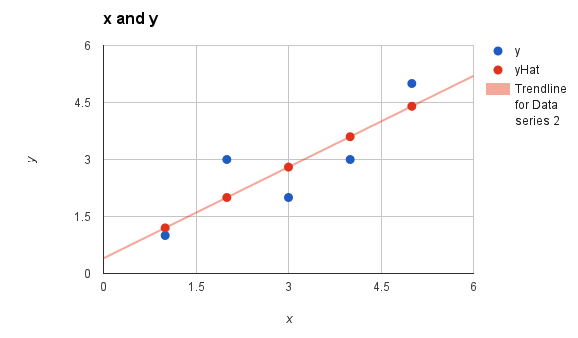# Explain linear regression

Linear regression

Simple linear regression: finding a relationship between an independent variable and a dependent variable
Multiple linear regression: Finding a linear relationship between multiple independent variables and dependent variables.

1 Like

Linear Regression is perhaps one of the most known and well understood algorithm in statistics and machine learning. Before going into linear regression let us first understand what is regression. Regression is a method of modeling a target value based on independent predictors. This method is mostly used for forecasting and finding out cause and effect relationship between variables. Regression techniques mostly differ based on the number of independent variables and the type of relationship between the independent and dependent variables.

Linear regression is a type of regression analysis where the number of independent variables is one and there is a linear relationship between the independent(x) and dependent(y) variable.

Equation is given as : y = a_0 + a_1 * x